# Logical Reasoning - Test 8

## 25 Questions MCQ Test SSC CGL Tier 1 Mock Test Series | Logical Reasoning - Test 8

Description
Attempt Logical Reasoning - Test 8 | 25 questions in 15 minutes | Mock test for SSC preparation | Free important questions MCQ to study SSC CGL Tier 1 Mock Test Series for SSC Exam | Download free PDF with solutions
QUESTION: 1

### Select the odd letters from the given alternatives.

Solution: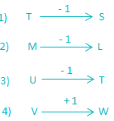→ In VW, W is the next alphabet from V, not previous.

QUESTION: 2

### Select the odd number from the given alternatives.

Solution:

→ if we observe carefully, we can see that the sum of digit of each number is 8 except 63.
2 + 6 = 8
4 + 4 = 8
6 + 3 = 9
3 + 5 = 8
Therefore, “63” is odd one among them.

QUESTION: 3

### Choose the odd word from the given alternatives.

Solution:

Quit India Movement, Non – Cooperation and Salt Satyagraha Movement were the movements led by Mahatama Gandhi but Bardoli Satyagraha Movement was led by Vallabhbhai Patel.

Therefore, “Bardoli Satyagraha Movement” is odd one among the given alternatives.

QUESTION: 4

Select the related letters from the given alternatives.
AD : N : : BD : ?

Solution:

Position of A = 1, D = 4 and N = 14 in English alphabets.
→ AD = N ⇒ 14 = 14
Similarly,
Position of B = 2, D = 4 and X = 24 in English alphabets.
→ BD = X ⇒ 24 = 24
Hence, “X” is the correct answer.

QUESTION: 5

Select the related word from the given alternatives.
Copper : ductile ∷ chalk : ?

Solution:

→ Ductility is the most important physical property of copper. Ductility of a material is its ability to deform when a force is applied upon it.
→ Brittleness is the physical property of chalk. Brittleness is exactly an opposite property of ductility as it is the ability of a material to break without first undergoing any kind of deformation.
→ In Brittleness, material breaks down without any deformation. Chalk material having such physical property therefore, chalk is related to brittle.

QUESTION: 6

In the following question, select the related number from the given alternatives.
2/3 : 19/29 : : 8/7 : ?

Solution:

;The pattern followed is
2/3 : 19/29
→ 2/3: (2 × 10 – 1)/(3 × 10 – 1)
Similarly,
8/7: (8 × 10 – 1)/(7 × 10 – 1)
Hence,  the missing term is 79/69.

QUESTION: 7

A series is given with one term missing. Select the correct alternative from the given ones that will complete the series.
2.5, 13/6, 11/6, _____, 7/6

Solution:

Sequence is obtained by following the rule given below: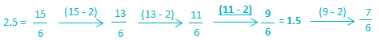Therefore, next term in series will be “1.5”

QUESTION: 8

In the given series one word is missing. Select the correct alternative from the given ones that will complete the series.
Cinnaba, barcode, decibel, elastic, ______________

Solution:

If we observe series carefully we can see that last two letter of each word is first two letter of next word.
Therefore, “iceberg” will be next term of series.

QUESTION: 9

Which set of letters when sequentially placed at the gaps in the given letter series shall complete it?
j __ l __ jk __ m __ kl __

Solution:

1) jkljm ⇒ j j l k jk l m j kl m
2) kmjjj ⇒ j k l m jk j m j kl j
3) kmljm ⇒ j k l m jk l m j kl → jklm/jklm/jklm
4) mkjlm ⇒ j m l k jk j m l kl m
Hence, “kmljm” will complete the series.

QUESTION: 10

How many points may be there on the face opposite to the face with 2 points?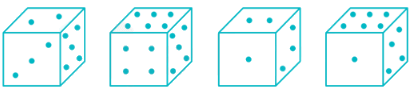Solution:

From figure 1st and 2nd points 2, 3, 4 and 6 are adjacent to 5.That means 1 is opposite to 5.
As 2 and 3 are adjacent to each other, out of 4 and 6, one will be opposite to 2.
Now from the given options we can say that 6 points may be there on the face opposite to the face with 2 points.

QUESTION: 11

Mithun travelled 12 kms southward, then turned left and travelled 10 kms, then turned left and travelled 12 km. How far was Mithun form the starting point?

Solution: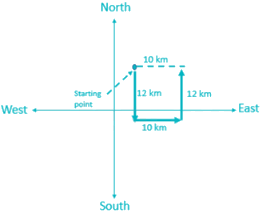Clearly, Mithun is 10 km far from starting point.

QUESTION: 12

A word is represented by only one set of numbers as given in any one of the alternatives. The sets of numbers given in the alternatives are represented by two classes of alphabets as shown in the given two matrices. The columns and rows of Matrix – I are numbered from 0 to 4 and that of Matrix – II are numbered from 5 to 9. A letter from these matrices can be represented first by its row and next by its column, for example, ‘N’ can be represented by 24, 66, etc. and ‘Q’ can be represented by 42, 97 etc. Similarly, you have to identify the set for the word ‘EAGER’.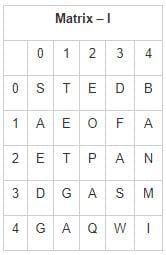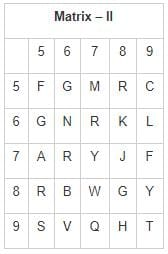Solution:

1) 02, 10, 65, 11, 68 ⇒ E, A, G, E, K
2) 02, 10, 65, 87, 85 ⇒ E, A, G, W, R
3) 02, 10, 65, 11, 85 ⇒ E, A, G, E, R
4) 02, 10, 65, 59, 85 ⇒ E, A, G, C, R
Hence, “02, 10, 65, 11, 85” are set of number which represent word “EAGER”

QUESTION: 13

Direction: Find which one word cannot be made from the letters of the given word.
REFRIGERATE

Solution:

There are only 3 ‘E’ in word REFEREE but there required 4 ‘E’ in word REFEREE.
Therefore, option 3) is correct answer.

QUESTION: 14

Which of the following diagrams indicates the best relation between snake, monkey and mammals?

Solution:

→ All mammals produce milk for their babies to drink. Monkey comes under mammal category but snakes are not mammals. So option 1st is correct.

QUESTION: 15

In a certain code language, "SUBSTITUTION" is written as "ITSBUSNOITUT". How is DISTRIBUTION written in that code language?

Solution:

SUBSTITUTION is written as ITSBUSNOITUT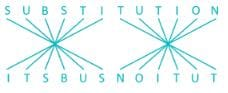Similarly,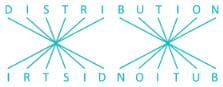DISTRIBUTION written as “IRTSIDNOITUB” in given code language.

QUESTION: 16

Find the missing term in diagram shown below.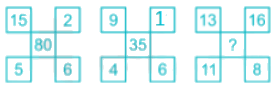Solution:

In first diagram,
⇒ (15 – 5) × (6 + 2) = 10 × 8 = 80
In Second diagram,
⇒ (9 – 4) × (1 + 6) = 5 × 7 = 35
In Third diagram,
⇒ (13 – 11) × (8 + 16) = 2 × 24 = 48
Therefore, “48” is missing term.

QUESTION: 17

Jais and his father has an age difference of 35 years now. After 5 years, the sum of their age is 125. What will be the age of Jais and his father after 12 years from now?

Solution:

Let the present age of Jais be X years and the age of his father be Y years.
Presently, Jais and his father has an age difference of 35 years.
→ Y – X = 35. ----------- (1)
After 5 years, the sum of their ages will be 125.
→ (X + 5) + (Y + 5) = 125
→ X + Y + 10 =125
→ Y + X = 115. ----------- (2)
Y – X + Y + X = 35 + 115
→ 2Y = 150
→ Y = 75.
Similarly, X = Y – 35 = 75 – 35 = 40.
After 12 years, Jais age is 52 (40 + 12) years and his father’s age will be 87 (75 + 12) years.
Hence, the correct answer is 52, 87.

QUESTION: 18

Some equation is solved on the basis of a certain system. Find the correct answer for the unsolved equation on the basis of solved equation.
58 × 12 = 4, 37 × 96 = 5,
11 × 20 = 2, 42 × 12 =?

Solution:

The given logic is as follows.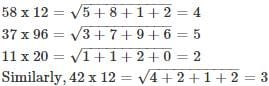QUESTION: 19

Select the correct combination of mathematical signs to replace * signs and to balance the following to form an equation:
( √121 * 9) * (5 * 4) * 1

Solution:

Let us first simplify the given equation:
( √121 * 9) * 5 * 4 * 1→ (11 * 9) *  5 * 4 * 1
Now we will check each option one by one:
(11 * 9) * 5 * 4 * 1→ (11 + 9) ÷ (5 × 4) = 20 ÷ (5 × 4)
Now if we take 20 ÷ (5 × 4) = 20 ÷ 20 = 1 True, thus this option can be correct.
(√121 * 9) * 5 * 4 * 1→ (11 = 9) + 5 × 4 ÷ 1 False, thus this option is wrong.
(11 * 9) * 5 * 4 * 1→ (11 – 9) × 5 + 4 = 1 False, thus this option is wrong.
(11 * 9) * 5 * 4 * 1→ (11 – 9) + 5 × 4 = 1False, thus this option is wrong.Hence possible answer is + ÷ × =.

QUESTION: 20

Eight people A, B, C, D, E, F, G and H are sitting around the circular table facing towards center of it. A and B are sitting opposite to each other. F is sitting immediate right of A. There is at least one person between B and H and H is not the neighbor of F. C and D are sitting opposite to each other and none of them is sitting adjacent to F.G is not sitting opposite to F.
Question: Who is sitting opposite to H?

Solution:

People are sitting around the circular table in the following way-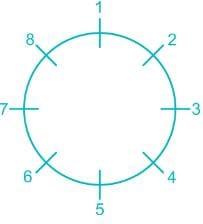All the eight positions are named from1 to 8.
Now it is told that, A and B are sitting opposite to each other and F is sitting immediate right of A.
So our arrangement will look like-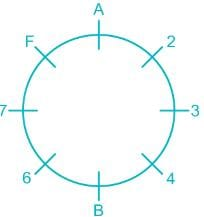Now There is at least one person between B and H and H is not the neighbor of F so H can sit at position 3, 4, or 6.
Now it is told that neither C or D is adjacent to F and C and D are opposite to each other,
Hence our arrangement will look like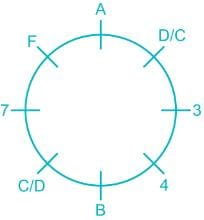So H has to take position 3.
Now it is told that G is not opposite to F then E will be opposite to F.
Then our final arrangement will look like-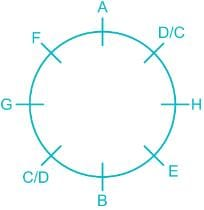Hence G is seating opposite to H.

QUESTION: 21

A piece of paper is folded and cut as shown below in the question figures. From the given answer figures, indicate how it will appear when opened.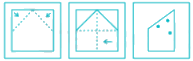Solution: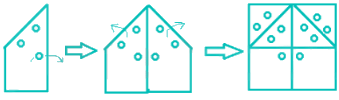One unfolding the punched paper, we get:
Thus option 3 is the correct option.

QUESTION: 22

Which answer figure will complete the pattern in the question figure?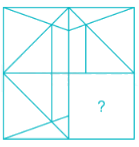Solution:

The missing part is option 1 as shown below.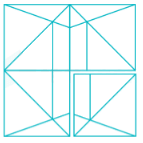QUESTION: 23

If the mirror is placed on the line AB, then which of the answer figure is the right image of the given figure?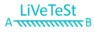Solution:

Here, the correct mirror image of above figure will be,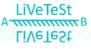Hence, the correct option is 4.

QUESTION: 24

Directions: In the question below are three statements followed by three conclusions numbered I, II and III. You have to take the three given statement to be true even if they seem to be at variance from commonly known facts and then decide which of the given conclusion logically follows from the three given statements, disregarding commonly known facts.
Statements:
Some rivers are hills.
No hills are taxi.
All taxi are buses.
Conclusions:
I. Some buses are rivers.
II. Some rivers are definitely not taxi.
III. No bus is river.

Solution:

From the statements, the least possible Venn diagram can be drawn as below: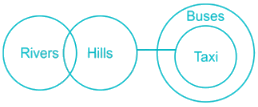Conclusions:
I. Some buses are rivers → Its possible but not definitely true, hence false.
II. Some rivers are definitely not taxis →Some rivers are hills and no hill is taxi, so that much portion of river which is hill will never be taxi. Hence true.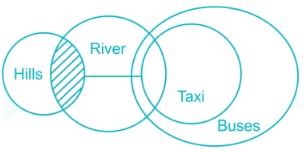III. No bus is river → Its possible but not definitely true.
Hence, only conclusion II follows.
But, since I and III make complementary pair hence, either I or III also follow.
So, conclusion II and either I or III follow.
Therefore, none of these is the correct option as there is no such option like conclusion II and either I or III follows.

QUESTION: 25

In the following question, two statements are given each followed by two conclusions I and II. You have to consider the statements to be true even if they seem to be at variance from commonly known facts. You have to decide which of the given conclusions, if any, follows from the given statements.
Statement:
(I) Women generally prefer window shopping.
(II) Males generally are not fond of window shopping and they feel that it is a sheer waste of time.
Conclusions:
(I) Shopping behavior is different for males and females.
(II) If males go for window shopping, they don't utilize much time in selecting a product.

Solution:

As in statement it is mentioned that Women prefer Window shopping and Males does not prefer. Hence, conclusion (I) follows as shopping behaviour is different for males and female.
But conclusion (II) does not follow as in statement nothing is mentioned if males go for windows shopping, they don’t utilize much time in selecting a product.
Hence, only conclusion I follows.Use Code STAYHOME200 and get INR 200 additional OFF Use Coupon Code